Derivation and Application of a Linear Multistep Numerical Scheme

Abdulrahman NDANUSA

Mathematics/Computer Science Department, Federal University of Technology, Minna, Nigeria

Abstract

A great many physical occurrences give rise to problems that often result in differential equations. When we solve a differential equation, we are in effect solving the physical problem it represents. Traditionally, solutions to differential equations were derived using analytical or exact methods. These solutions are often useful as they provide excellent insight into the behavior of some systems. However, certain differential equations are very difficult to solve by any means other than an approximate solution by the application of numerical methods. These methods can be classified into two thus: One-step and multistep methods. However, in this work, our focus is on a class of multistep methods known as the Linear Multi-step Methods. We thus use Taylor series expansion to derive a linear multistep method of order eight. The method is tested for consistency and zero-stability in order to establish its convergence. Also, provided are some examples of problems solved using the new scheme, and the results compared with exact solution.

Introduction

We consider the initial value problem (IVP):

 y' = f(t,y),                     y(t0) = y0,                     t > t0 (1)

Let yn be an approximation to the theoretical solution at tn, that is, to y(tn), and let fn=f(tn,yn). Then, we say a linear multistep method (LMM) of step number k, or a linear k -step method is a computational method for determining, the sequence {yn} that takes the form of a linear relationship between yn+j, fn+j, j = 0,1, …, k.

Thus the general lmm may be written: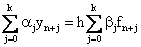(2)

where αj and βj are constants; we assume αj = 1 and that not both α0 and β0 are zero. We say that the method is explicit if βk = 0, and implicit if βk ≠ 0 .

Convergence is a minimal property which any acceptable linear multistep method must possess. Thus, the necessary and sufficient conditions for a linear multistep method to be convergent are that it be consistent and zero-stable .

Consistency demands that,

 ρ(1) = 0           and       ρ'(1) = σ(1) (3)

where,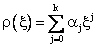(4)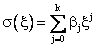(5)

are called the first and second characteristic polynomials of (2) respectively.

The lmm (2) is said to be zero-stable if no root of (4) has modulus greater than one, and if every root with modulus one is simple .

Derivation of the Lmm of Order 8

We use the method of Taylor series expansion described by  as follows:

Let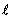be the linear difference operator defined by: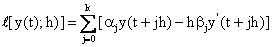(6)

where y(t) is an arbitrary function, continuously differentiable on [a , b].

Suppose we choose to expand y(t+jh) and y(t+jh) about t+rh; where r need not necessarily be an integer. We obtain: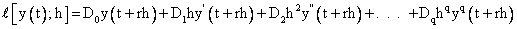(7)

The formulae for the constants Dq expressed in terms of αj, βj are:(8)

q = 2, 3, …

In this research work, we wish to derive an optimal 6-step method. Therefore, all the roots of the first characteristic polynomial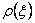must be on the unit circle. We know thatis a polynomial of degree 6. Hence, by consistency, it has one real root at +1 and another real root at -1. The four remaining roots must be complex.

Hence we have:

 ξ1 = +1 , ξ2 = -1, ξ3 = eiθ1, ξ3 = e-iθ1, ξ3 = eiθ2, ξ3 = e-iθ2

Hence:

 ρ(ξ) = (ξ - 1)(ξ + 1)(ξ - eiθ1)(ξ - e-iθ1)(ξ - eiθ2) (ξ-e-iθ2) α6 = +1, α5 = -2(a+b), α4 = (4ab + 1), α3 = 0, α2 = -(4ab+1), α1 = 2(a+b), α0 = -1

We require the method to have order 8. We now state the order requirement in terms of the coefficients Dq .

From (8) we have the following:

 D0 = α0 + α1 + α2 + α3 + α4 + α5 + α6 D1 = -rα0 + (1 - r)α1 + (2 - r)α2 + (3 - r)α3 + (4 - r)α4 + (5 - r)α5 + (6 - r)α6 -          - (β0 + β1 + β2 + β3 + β4 + β5 + β6) D2 = 1/2![(- r)2α0 + (1 - r)2α1 + (2 - r)2α2 + (3 - r)2α3 + (4 - r)2α4 + (5 - r)2α5 +          + (6 - r)2α6] - [-rβ0 + (1 - r)β1 + (2- r)β2 + (3 - r)β3 + (4 - r)β4 + (5 - r)β5 +          + (6 - r)β6] . . . D8 = 1/8![(- r)8α0 + (1 - r)8α1 + (2 - r)8α2 + (3 - r)8α3 + (4 - r)8α4 + (5 - r)8α5 +          + (6 - r)8α6] - 1/7![(-r) 7β0 + (1 - r)7β1 + (2- r) 7β2 + (3 - r) 7β3 + (4-r) 7β4 +          + (5 - r) 7β5 + (6 - r) 7β6] D9 = 1/9![(- r)9α0 + (1 - r)9α1 + (2 - r)9α2 + (3 - r)9α3 + (4 - r)9α4 + (5 - r)9α5 +          + (6 - r)9α6] - 1/8![(-r)8β0 + (1 - r)8β1 + (2- r)8β2 + (3 - r)8β3 + (4 - r)8β4 +          + (5 - r)8β5 + (6 - r)8β6]

Setting r = 3 and Dq = 0, q = 2, 3, 4, 5, 6, 7, 8 we have:

 D2 = 1/2![32α0 +22α1 + α2 + α4 + 22α5 + 32α6] -          - [-3β0 - 2β1 - β2 + β4 + 2β5 + 3β6] = 0 D3 = 1/3![-33α0 - 23α1 - α2 + α4 + 23α5 + 33α6] -          - 1/2![32β0 + 22β1 + β2 + β4 + 22β5 + 32β6] = 0 D4 = 1/4![34α0 + 24α1 + α2 + α4 + 24α5 + 34α6] -          - 1/3![-33β0 - 23β1 - β2 + β4 + 23β5 + 33β6] = 0 D5 = 1/5![-35α0 - 25α1 - α2 + α4 + 25α5 + 35α6] -          - 1/4![34β0 + 24β1 + β2 + β4 + 24β5 + 34β6] = 0 D6 = 1/6![36α0 + 26α1 + α2 + α4 + 26α5 + 36α6] -          - 1/5![-35β0 - 25β1 - β2 + β4 + 25β5 + 35β6] = 0 D7 = 1/7![-37α0 - 27α1 - α2 + α4 + 27α5 + 37α6] -          - 1/6![36β0 + 26β1 + β2 + β4 + 26β5 + 36β6] = 0 D8 = 1/8![38α0 + 28α1 + α2 + α4 + 28α5 + 38α6] -          - 1/7![-37β0 - 27β1 - β2 + β4 + 27β5 + 37β6] = 0

However, on inserting the values we have obtained for the αj into these equations we have:

 -3β0 - 2β1 - β2 + β4 + 2β5 + 3β6] = 0 32β0 + 22β1 + β2 + β4 + 22β5 + 32β6 = 2/3[28 + 4ab - 16(a + b)] -33β0 - 23β1 - β2 + β4 + 23β5 + 33β6 = 0 34β0 + 24β1 + β2 + β4 + 24β5 + 34β6 = 2/5[244 + 4ab - 64(a + b)] -35β0 - 25β1 - β2 + β4 + 25β5 + 35β6 = 0 36β0 + 26β1 + β2 + β4 + 26β5 + 36β6 = 2/7[2188 + 4ab - 256 (a + b)] -37β0 - 27β1 - β2 + β4 + 27β5 + 37β6 = 0 (9)

We can satisfy the first, third, fifth and seventh of these equations if we choose: β2 = β4, β1 = β5, β0 = β6. The remaining three equations give:

 32 β0 + 22 β1 + β2 = 1/3[28 + 4ab - 16(a+b)] (10) 34 β0 + 24 β1 + β2 = 1/5[244 + 4ab - 64(a+b)] (11) 36 β0 + 26 β1 + β2 = 1/7[2188 + 4ab - 256(a+b)] (12)

From (10) we have:

 β2 = 1/3[28 + 4ab - 16(a+b)] - 9β0 - 4 β1 (13)

Substituting (13) into (11):

 81β0 + 16β1 + 1/3[28 + 4ab - 16(a+b)] - 9β0 - 4 β1 = 1/5[244 + 4ab - 64(a+b)] 72β0 + 12β1 = 1/5[244 + 4ab - 64(a+b)] - 1/3[28 + 4ab - 16(a+b)] 72β0 + 12β1 = 1/15[592 - 8ab - 112(a+b)] (14)

Substituting (13) into (12):

 729β0 + 64β1 + 1/3[28 + 4ab - 16(a+b)] - 9β0 - 4 β1 = 1/7[2188 + 4ab - 256(a+b)] 720β0 + 60β1 = 1/7[2188 + 4ab - 256(a+b)] - 1/3[28 + 4ab - 16(a+b)] 720β0 + 60β1 = 1/21[6368 + 4ab - 656(a+b)] (15)

From (14) and (15), solving simultaneously to have:

 β0 = 1/945[278 + 5ab + 16(a+b)] = β6 (16)

Substituting (16) into (14), we have:

 β1 = 1/150[160 - 8ab - 76(a+b)] = β5 (17)

Substituting (16) and (17) into (13) gives:

 β2 = 1/105[62 + 167ab - 272(a+b)] = β4 (18)

Finally, solving D1 = 0 gives:

 β 3 = 1/945[3008 + 5688ab - 1328(a+b)] (19)

We solve for the error constant, D9:

 D9 = 1/9![-39α0 - 29α1 - α2 + α4 + 29α5 + 39α6] -          - 1/8![38β0 + 28β1 + β2 + β4 + 28β5 + 38β6] D9 = -1/907200[6016 + 736ab - 8576(a+b)] (20)

Since a = cosθ1, b = cosθ2, 0 < θ1 < π, 0 < θ2 < π, a and b are restricted to the range -1 < a < 1 and -1 < b < 1. Our choice of values for a and b is guided by the fact that we like to minimize the error constant as well as the need to develop a method that makes computation easier by reducing the number of operations involved.

The following values are therefore, assigned to the variables: a = 3/4, b = -1/3.

Hence the following values are obtained for the coefficients αi, βi:

 α6 = + 1, α5 = - 5/6, α4 = 0, α3 = 0, α2 = 0, α1 = 5/6, α0 = - 1 β0 = 3401/11340 = β6, β1 = 391/315 = β5, β2 = -1117/1260 = β4, β1 = 3848/2835

Substituting the values of a and b into (20), the Error constant is: -0.002489711924.

And finally, we have the scheme:

 yn+6 -5/6 yn+5 +5/6 yn+1 - yn = = h[(3401/11340)fn+6 + (391/315)fn+5 - (1117/1260)fn+4 + (3848/2835)fn+3 -    - (1117/1260)fn+2 + (391/315)fn+1 + (3401/11340)fn] (21)

Test for Convergence

To prove that the scheme converges, it is sufficient for us to show that it is consistent as well as zero-stable.

To prove consistency, we have from (3), (4) and (5):

 ρ(1) = 1 - 5/6 + 5/6 - 1 = 0 (22) ρ'(1) = 6(1) - 5(5/6) + 1(5/6) - 0(0) = 2.666666667 (23) σ(1) = 2.666666667 (24)

Equations (22) - (24) establish consistency.

For zero-stability, we consider the following equation that represents the first characteristic polynomial of the scheme:

 ρ(ξ) = ξ6 - 5/6 ξ5 5 5/6 ξ - 1 (25)

The following are the roots of (25):

 ξ1 = + 1, ξ2 = - 1, ξ3 = [(3 + Ö7)/4]i, ξ4 = [(3 - Ö7)/4]i, ξ5 = [(-1 +2Ö2)/3]i, ξ6 = [(-1 - 2Ö2)/3]i

It is obvious that | ξ1| ≤ 1, I = 1, 2, 3, 4, 5, 6. Thus ζi = 1, 2, …, 6 satisfy the zero stability condition. Hence, we conclude that scheme 1 is convergent.

Numerical Examples

We use the scheme to solve some differential equations as follows. However, as with all k-step methods (k < 1) we need to use another method to calculate additional starting values. In this work, we decide to use the Fourth Order Runge-Kutta method to evaluate the starting values yn, n = 0, 1, …, 5, since the Runge-Kutta methods constitute the most efficient method for generating starting values for linear multistep methods.

The Fourth order Runge-Kutta method is given below:

 yn+1 = yn + h/6(k1 + 2k2 + 2k3 + k4) k1 = f(xn, yn), k2 = f(xn +1/2h, yn + 1/2hk1), k3 = f(xn +1/2h, yn + 1/2hk2), k4 = f(xn +h, yn + hk1)

We choose as our predictor, the fourth order Adams-Bashforth method:

 yn + 4 = yn + 3 +h/24(55fn+3 - 59fn+2 + 37fn+1 - 9fn)

Discussion of Results

In Table 1 the scheme produced errors as shown, within acceptable limits. The scheme is very accurate as exhibited in Table 2; there is no error at all. This is understandable because the solution of the differential equation is a polynomial of degree three, i.e., not greater than six, the step number.

Conclusion

Since we have used the numerical method to solve various differential equations we conclude that the method is accurate as it produced results which are comparable with those produced by other similar methods.

Table 1. Problem: F=X+Y; Y(0)=1; h=0.1; exact solution: Y(X)=2*EXP(X)-X-1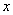Exact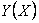Error 0.0 1.0000000000 1.0000000000 0.0000000000E+00 0.1 1.1103418362 1.1103416667 1.6948462878E-07 0.2 1.2428055163 1.2428051417 3.7461895075E-07 0.3 1.3997176152 1.3997169941 6.2102693121E-07 0.4 1.5836493953 1.5836484802 9.1512116951E-07 0.5 1.7974425414 1.7974412772 1.2642065803E-06 0.6 2.0442376008 2.0442361876 1.4132161703E-06 0.7 2.3275054149 2.3275025204 2.8945628872E-06 0.8 2.6510818570 2.6510783589 3.4980860688E-06 0.9 3.0192062223 3.0192004614 5.7608878645E-06 1.0 3.4365636569 3.4365566462 7.0107547572E-06

Table 2. Problem: F=3X2-6X+5; Y(0)=1; h=0.1; Exact Solution: Y(X)=X3-3X2+5X+1

Reference

1.         Lambert j. d., Computational Methods in Ordinary Differential Equations, John Wiley, 1973, p. 12 & 33& 38.